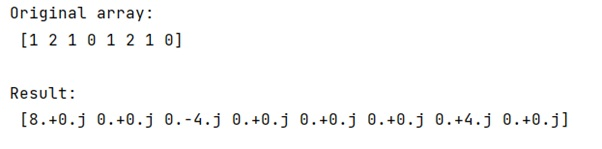# How does numpy.fft.fft() work?

Learn about the Python's numpy.fft.fft() method, and its usages with examples.
Submitted by Pranit Sharma, on February 16, 2023

NumPy is an abbreviated form of Numerical Python. It is used for different types of scientific operations in python. Numpy is a vast library in python which is used for almost every kind of scientific or mathematical operation. It is itself an array which is a collection of various methods and functions for processing the arrays.

## Python | numpy.fft.fft() Method

The numpy.fft.fft() method computes the one-dimensional discrete Fourier Transform. This function computes the one-dimensional n-point discrete Fourier Transform (DFT) with the efficient Fast Fourier Transform (FFT) algorithm [CT].

Syntax:

```numpy.fft.fft(a, n=None, axis=-1, norm=None)
```

Parameter(s):

• a: array_like - The input array, can be complex.
• n: int, optional - Length of the transformed axis of the output. If n is smaller than the length of the input, the input is cropped. If it is larger, the input is padded with zeros. If n is not given, the length of the input along the axis specified by the axis is used.
• axis: int, optional - Axis over which to compute the FFT. If not given, the last axis is used.
• norm: {"backward", "ortho", "forward"}, optional.

Let us understand with the help of an example,

## Python code to demonstrate the example of numpy.fft.fft() method

```# Import numpy
import numpy as np

# Creating a numpy array
arr = np.array([1,2,1,0,1,2,1,0])

# Display original array
print("Original array:\n",arr,"\n")

# Computing fft values
res = np.fft.fft(arr)

# Display Result
print("Result:\n",res)
```

Output: